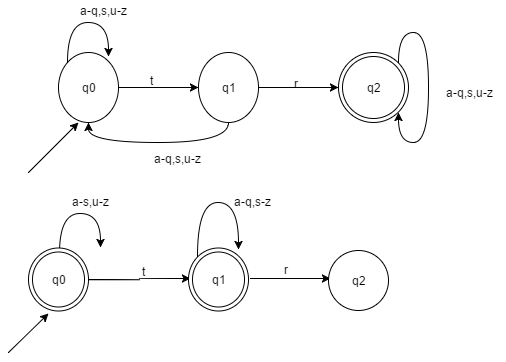## Automata Theory Questions and Answers Part-9

1. Which of the following recognizes the same formal language as of DFA and NFA?
a) Power set Construction
b) Subset Construction
c) Robin-Scott Construction
d) All of the mentioned

Explanation: All the three option refers to same technique if distinguishing similar constructions for different type of automata.

2. Under which of the following operation, NFA is not closed?
a) Negation
b) Kleene
c) Concatenation
d) None of the mentioned

Explanation: NFA is said to be closed under the following operations:
a) Union
b) Intersection
c) Concatenation
d) Kleene
e) Negation

3. It is less complex to prove the closure properties over regular languages using
a) NFA
b) DFA
c) PDA
d) Can’t be said

Explanation: We use the construction method to prove the validity of closure properties of regular languages. Thus, it can be observe, how tedious and complex is the construction of a DFA as compared to an NFA with respect to space.

4. Which of the following is an application of Finite Automaton?
a) Compiler Design
b) Grammar Parsers
c) Text Search
d) All of the mentioned

Explanation: There are many applications of finite automata, mainly in the field of Compiler Design and Parsers and Search Engines.

5. John is asked to make an automaton which accepts a given string for all the occurrence of ‘1001’ in it. How many number of transitions would John use such that, the string processing application works?
a) 9
b) 11
c) 12
d) 15

Explanation:6. Which of the following do we use to form an NFA from a regular expression?
a) Subset Construction Method
b) Power Set Construction Method
c) Thompson Construction Method
d) Scott Construction Method

Explanation: Thompson Construction method is used to turn a regular expression in an NFA by fragmenting the given regular expression through the operations performed on the input alphabets.

7. Which among the following can be an example of application of finite state machine(FSM)?
c) Stack
d) None of the mentioned

Explanation: Idle is the state when data in form of packets is send and returns if NAK is received else waits for the NAK to be received.

8. Which among the following is not an application of FSM?
a) Lexical Analyser
b) BOT
c) State charts
d) None of the mentioned

Explanation: Finite state automation is used in Lexical Analyser, Computer BOT (used in games), State charts, etc.

9. L1= {w | w does not contain the string tr }
L2 = {w | w does contain the string tr}
Given ∑ = {t, r}, The difference of the minimum number of states required to form L1 and L2?
a) 0
b) 1
c) 2
d) Cannot be said